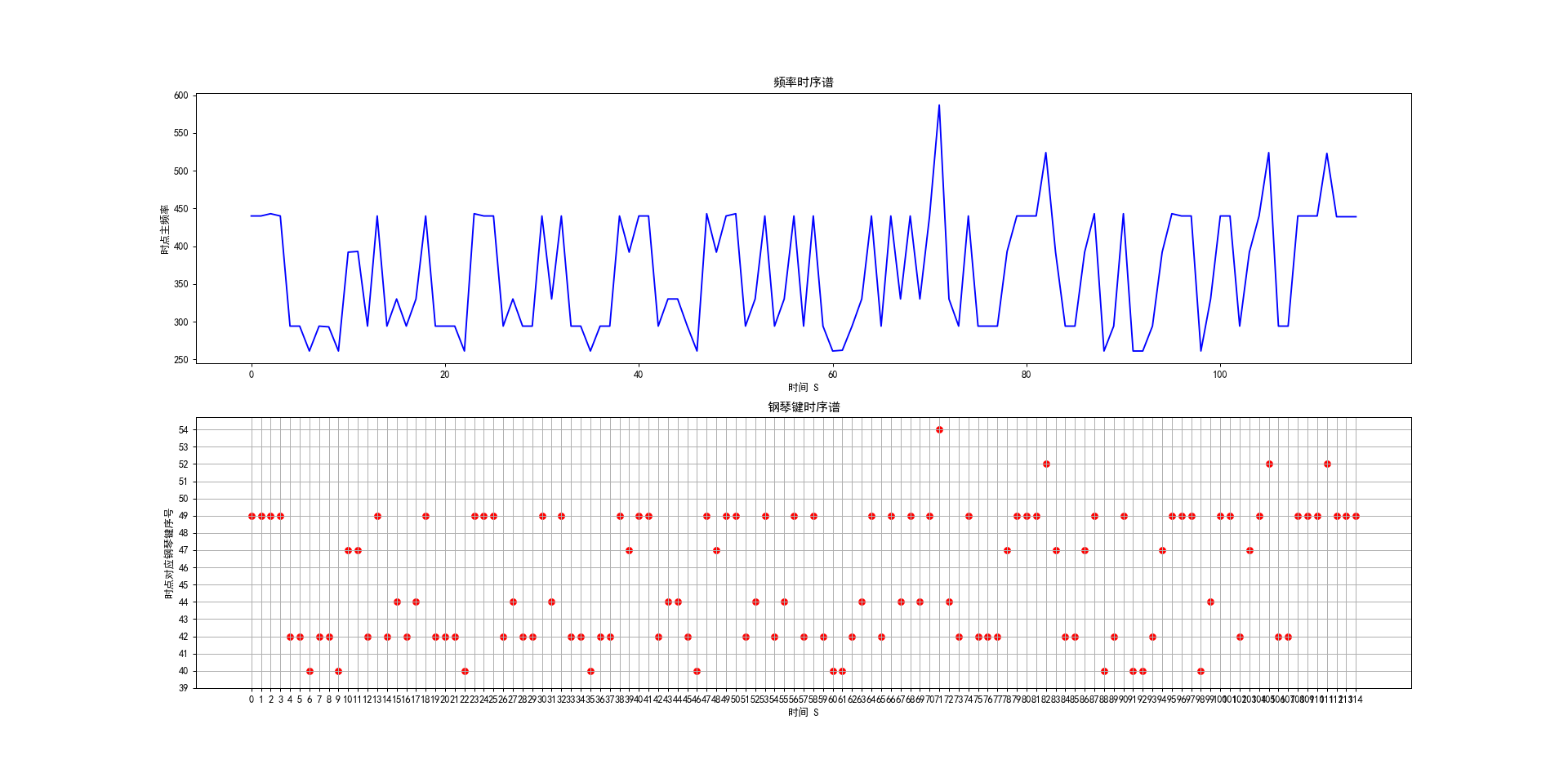• VC实现FIR数字信号滤波,可以分析wav文件的频谱和滤波后的频谱
• Wav信号的录制与频谱分析 程序结构 #mermaid-svg-gx3gxXF0z0hCXRDi .label{font-family:'trebuchet ms', verdana, arial;font-family:var(--mermaid-font-family);fill:#333;color:#333}#mermaid-svg-gx3gxXF0z0...
##matlab转python实现（四）
##后附完整代码及windows环境下的pyaudio库的安装。
Wav信号的录制与频谱分析
程序结构

:root { --mermaid-font-family: "trebuchet ms", verdana, arial;}#mermaid-svg-gx3gxXF0z0hCXRDi {
color: rgba(0, 0, 0, 0.75);
font: ;
}录制Wav音频读取音频信号时域波形频域波形
自定义函数
#定义sound recoding 函数
参数为录音时间t。
def sound_rec(t):

#定义wav文件读取函数
参数为wav文件的路径path,代码中因为保存的录音文件就在该代码路径下，可以不用输入绝对路径。返回值为:左右声道的波形数组wave_data,采样率framerate，采样率*时间nframes
def wave_read(path):

#定义画出时域上的波形图函数time plot
第一个参数为采样率，第二个参数为采样率*时间,第三个参数为左右声道的数据wave data list
def time_plt(frames,nframes,wave):

#定义画出信号频谱上的波形图函数frequence plot
第一个参数为采样率，第二个参数为左右声道的数据wave data list
def freq_plt(frames,wave):

主要进程
a.
录制音频，将音频保存在文件路径下的output.wav文件中。(本步骤可以忽略，在已经录制过音频的情况下。)
b.
读取音频信号，将双声道信号的信息存储在数组中，并得出采样率，以及信号长度。
c.
做出时域上的波形图，频域上，进行傅里叶变换，单边谱处理以及归一化处理，得出频域幅度谱，最后一起显示。
附完整代码
import wave
import pyaudio
import numpy
from pyaudio import PyAudio
import matplotlib.pyplot as plt

#定义sound recoding 函数,其参数为录音时间t
def sound_rec(t):
# 定义数据流块
CHUNK = 1024
FORMAT = pyaudio.paInt16
CHANNELS = 2
RATE = 44100
# 录音时间
RECORD_SECONDS = t
# 要写入的文件名
WAVE_OUTPUT_FILENAME = "output.wav"
# 创建PyAudio对象
p = pyaudio.PyAudio()
# 打开数据流
stream = p.open(format=FORMAT,channels=CHANNELS,rate=RATE,input=True,frames_per_buffer=CHUNK)
print("start recording")
# 开始录音
frames = []
for i in range(0, int(RATE / CHUNK * RECORD_SECONDS)):
frames.append(data)
print("done recording")
# 停止数据流
stream.stop_stream()
stream.close()
# 关闭PyAudio
p.terminate()
# 写入录音文件
wf = wave.open(WAVE_OUTPUT_FILENAME, 'wb')
wf.setnchannels(CHANNELS)
wf.setsampwidth(p.get_sample_size(FORMAT))
wf.setframerate(RATE)
wf.writeframes(b''.join(frames))
wf.close()

#定义wav文件读取函数，其参数为wav文件的路径path
#返回值为:左右声道的波形数组wave_data,采样率framerate，采样率*时间nframes
wf = wave.open(path, 'rb')
# 创建PyAudio对象
p = PyAudio()
stream = p.open(format = p.get_format_from_width(wf.getsampwidth()),
channels = wf.getnchannels(),
rate = wf.getframerate(),
output = True)
nframes = wf.getnframes()
framerate = wf.getframerate()
# 读取完整的帧数据到str_data中，这是一个string类型的数据
wf.close()
# 将波形数据转换成数组
wave_data = numpy.fromstring(str_data, dtype=numpy.short)
# 将wave_data数组改为2列，行数自动匹配
wave_data.shape = -1,2
# 将数组转置
wave_data = wave_data.T
return wave_data,framerate,nframes

#定义画出时域上的波形图函数time plot
#第一个参数为采样率，第二个参数为采样率*时间,第三个参数为左右声道的数据wave data list
def time_plt(frames,nframes,wave):
# time也是一个数组，与wave_data或wave_data配对形成系列点坐标
time = numpy.arange(0, nframes)*(1.0/frames)
# 绘制波形图
plt.figure(num=1,figsize=(6,4))
plt.subplot(211)
plt.plot(time, wave, c='r')
plt.subplot(212)
plt.plot(time, wave, c='g')
plt.xlabel('time (seconds)')
plt.ylabel('ampliude')

#定义画出信号频域上的波形函数frequence plot
#第一个参数为采样率，第二个参数为左右声道的数据wave data list
def freq_plt(frames,wave):
# 采样点数，修改采样点数和起始位置进行不同位置和长度的音频波形分析
N = 44100
start = 0  # 开始采样位置
df = frames/(N-1)  # 分辨率
freq = [df*n for n in range(0, N)]  # N个元素
wave_data2 = wave[start:start+N]
c = numpy.fft.fft(wave_data2)*2/N
# 常规显示采样频率一半的频谱
d = int(len(c)/2)
# 仅显示频率在4000以下的频谱
while freq[d] > 4000:
d -= 10
plt.figure(num=2,figsize=(6,4))
plt.plot(freq[:d-1], abs(c[:d-1]), 'r')

#sound_rec(5)
time_plt(frames,nframes,wave)
freq_plt(frames,wave)
plt.show()

windows下安装pyaudio库
建议工具：一个python编辑器（用来查看你的python.exe存放的位置），windows powershell。
首先下载一个
直接使用pip install来安装会报错error：failed building wheel for pyaudio。
找适合自己的版本，本机环境为window+python3.7选择了PyAudio-0.2.11-cp37-cp37m-win_amd64.whl。自己看情况选择。
下载完后放在自己的python的Scripts文件夹下；这里找不到可以在自己的python编辑器里面找路径，会方便一点。
之后通过powershell进入scripts文件夹下:
~$: cd Scripts ~/Scripts$:  pip install  PyAudio-0.2.11-cp37-cp37m-win_amd64.whl
~/Scripts$: pip install pyaudio  最后试用上述代码，没报错就为成功。  展开全文python • Wav信号的录制与频谱分析程序结构录制Wav音频读取音频信号时域波形频域波形自定义函数#定义sound recoding 函数参数为录音时间t。def sound_rec(t):#定义wav文件读取函数参数为wav文件的路径path,代码中因为保存的... ##matlab转python实现(四)##后附完整代码及windows环境下的pyaudio库的安装。Wav信号的录制与频谱分析程序结构录制Wav音频读取音频信号时域波形频域波形自定义函数#定义sound recoding 函数参数为录音时间t。def sound_rec(t):#定义wav文件读取函数参数为wav文件的路径path,代码中因为保存的录音文件就在该代码路径下，可以不用输入绝对路径。返回值为:左右声道的波形数组wave_data,采样率framerate，采样率*时间nframesdef wave_read(path):#定义画出时域上的波形图函数time plot第一个参数为采样率，第二个参数为采样率*时间,第三个参数为左右声道的数据wave data listdef time_plt(frames,nframes,wave):#定义画出信号频谱上的波形图函数frequence plot第一个参数为采样率，第二个参数为左右声道的数据wave data listdef freq_plt(frames,wave):主要进程a.录制音频，将音频保存在文件路径下的output.wav文件中。(本步骤可以忽略，在已经录制过音频的情况下。)b.读取音频信号，将双声道信号的信息存储在数组中，并得出采样率，以及信号长度。c.做出时域上的波形图，频域上，进行傅里叶变换，单边谱处理以及归一化处理，得出频域幅度谱，最后一起显示。附完整代码import waveimport pyaudioimport numpyfrom pyaudio import PyAudioimport matplotlib.pyplot as plt#定义sound recoding 函数,其参数为录音时间tdef sound_rec(t):# 定义数据流块CHUNK = 1024FORMAT = pyaudio.paInt16CHANNELS = 2RATE = 44100# 录音时间RECORD_SECONDS = t# 要写入的文件名WAVE_OUTPUT_FILENAME = "output.wav"# 创建PyAudio对象p = pyaudio.PyAudio()# 打开数据流stream = p.open(format=FORMAT,channels=CHANNELS,rate=RATE,input=True,frames_per_buffer=CHUNK)print("start recording")# 开始录音frames = []for i in range(0, int(RATE / CHUNK * RECORD_SECONDS)):data = stream.read(CHUNK)frames.append(data)print("done recording")# 停止数据流stream.stop_stream()stream.close()# 关闭PyAudiop.terminate()# 写入录音文件wf = wave.open(WAVE_OUTPUT_FILENAME, 'wb')wf.setnchannels(CHANNELS)wf.setsampwidth(p.get_sample_size(FORMAT))wf.setframerate(RATE)wf.writeframes(b''.join(frames))wf.close()#定义wav文件读取函数，其参数为wav文件的路径path#返回值为:左右声道的波形数组wave_data,采样率framerate，采样率*时间nframesdef wave_read(path):wf = wave.open(path, 'rb')# 创建PyAudio对象p = PyAudio()stream = p.open(format = p.get_format_from_width(wf.getsampwidth()),channels = wf.getnchannels(),rate = wf.getframerate(),output = True)nframes = wf.getnframes()framerate = wf.getframerate()# 读取完整的帧数据到str_data中，这是一个string类型的数据str_data = wf.readframes(nframes)wf.close()# 将波形数据转换成数组wave_data = numpy.fromstring(str_data, dtype=numpy.short)# 将wave_data数组改为2列，行数自动匹配wave_data.shape = -1,2# 将数组转置wave_data = wave_data.Treturn wave_data,framerate,nframes#定义画出时域上的波形图函数time plot#第一个参数为采样率，第二个参数为采样率*时间,第三个参数为左右声道的数据wave data listdef time_plt(frames,nframes,wave):# time也是一个数组，与wave_data或wave_data配对形成系列点坐标time = numpy.arange(0, nframes)*(1.0/frames)# 绘制波形图plt.figure(num=1,figsize=(6,4))plt.subplot(211)plt.plot(time, wave, c='r')plt.subplot(212)plt.plot(time, wave, c='g')plt.xlabel('time (seconds)')plt.ylabel('ampliude')#定义画出信号频域上的波形函数frequence plot#第一个参数为采样率，第二个参数为左右声道的数据wave data listdef freq_plt(frames,wave):# 采样点数，修改采样点数和起始位置进行不同位置和长度的音频波形分析N = 44100start = 0 # 开始采样位置df = frames/(N-1) # 分辨率freq = [df*n for n in range(0, N)] # N个元素wave_data2 = wave[start:start+N]c = numpy.fft.fft(wave_data2)*2/N# 常规显示采样频率一半的频谱d = int(len(c)/2)# 仅显示频率在4000以下的频谱while freq[d] > 4000:d -= 10plt.figure(num=2,figsize=(6,4))plt.plot(freq[:d-1], abs(c[:d-1]), 'r')#sound_rec(5)wave,frames,nframes=wave_read('output.wav')time_plt(frames,nframes,wave)freq_plt(frames,wave)plt.show()windows下安装pyaudio库建议工具：一个python编辑器(用来查看你的python.exe存放的位置)，windows powershell。首先下载一个直接使用pip install来安装会报错error：failed building wheel for pyaudio。找适合自己的版本，本机环境为window+python3.7选择了PyAudio-0.2.11-cp37-cp37m-win_amd64.whl。自己看情况选择。下载完后放在自己的python的Scripts文件夹下；这里找不到可以在自己的python编辑器里面找路径，会方便一点。之后通过powershell进入scripts文件夹下:~$: cd Scripts~/Scripts$: pip install PyAudio-0.2.11-cp37-cp37m-win_amd64.whl~/Scripts$:  pip install pyaudio最后试用上述代码，没报错就为成功。
展开全文• 信号与系统：用matlab分析wav音频的频谱2018-11-25【天水浪客一门课程的平时作业，matlab确实强大，用它做这个有点杀鸡用牛刀的意味...软件太大了，直接靠安装了此软件的同学搞定，程序也是参考网上的资料... 惭愧 ....
信号与系统：用matlab分析wav音频的频谱2018-11-25【天水浪客一门课程的平时作业，matlab确实强大，用它做这个有点杀鸡用牛刀的意味...软件太大了，直接靠安装了此软件的同学搞定，程序也是参考网上的资料... 惭愧 ... 但总算是弄完了。发上来供后人借鉴吧 ...matlab7下载(1.01G)：http://www.21ft.com/ftpsite/incoming/matlab7.ISO1、下载后用虚拟光驱安装(天水浪客补：别弱到看成压缩包了)。2、序列号是13-22955-32678-26249-06848-40366-39620-03472-09765-20949-30945-19673-43738-38950-445483、关于matlab自动关闭的问题：这个问题是和CPU有关系,MATLAB要用到CPU的数学计算模块,而MATLAB默认的是INTEL的CPU,所以速龙或者是闪龙的CPU一般都会遇到安装好后打开自动关闭的问题.解决办法:先确认安装好后(假如安装在D盘)在此路径下D:\MATLAB7\bin\win32有一个叫做atlas_Athlon.dll的文件.然后在我的电脑上右击点"属性",再在"高级"中点"环境变量",在"系统变量"中点击"新建"输入以下信息: 变量名:BLAS_VERSION变量址:D:\MATLAB7\bin\win32\atlas_Athlon.dll】分析一个.wav文件的频谱分析的是ZR33.wav程序：[y,Fs,bits]=wavread("ZR33.wav");y=y(:,1);sigLength=length(y);Y = fft(y,sigLength);Pyy = Y.* conj(Y) / sigLength;halflength=floor(sigLength/2);f=Fs*(0:halflength)/sigLength;figure;plot(f,Pyy(1:halflength+1));xlabel("Frequency(Hz)");t=(0:sigLength-1)/Fs;figure;plot(t,y);xlabel("Time(s)");免责声明：本文仅代表文章作者的个人观点，与本站无关。其原创性、真实性以及文中陈述文字和内容未经本站证实，对本文以及其中全部或者部分内容文字的真实性、完整性和原创性本站不作任何保证或承诺，请读者仅作参考，并自行核实相关内容。http://www.pinlue.com/style/images/nopic.gif
展开全文• import wave import numpy from pyaudio import PyAudio import matplotlib.pyplot as plt from matplotlib.ticker ...wf = wave.open('e:/tests/audio2miditest.wav', 'rb') # 创建PyAudio对象 p = PyAudio() str...import wave
import numpy
from pyaudio import PyAudio
import matplotlib.pyplot as plt
from matplotlib.ticker import MultipleLocator, FormatStrFormatter
import math

wf = wave.open('e:/tests/audio2miditest.wav', 'rb')
# 创建PyAudio对象
p = PyAudio()
stream = p.open(format = p.get_format_from_width(wf.getsampwidth()),
channels = wf.getnchannels(),
rate = wf.getframerate(),
output = True)
nframes = wf.getnframes()
print("nframes",nframes)
framerate = wf.getframerate()

# 读取完整的帧数据到str_data中，这是一个string类型的数据
wf.close()

# 将波形数据转换成数组
wave_data = numpy.fromstring(str_data, dtype=numpy.short)
# 将wave_data数组改为2列，行数自动匹配
wave_data.shape = -1,2
# 将数组转置
wave_data = wave_data.T
print("wave_data shape",wave_data.shape)

#读取片段数
nsegments=int(wave_data.shape/framerate)
print("nsegments",nsegments)

def freqs():
# 采样点数，修改采样点数和起始位置进行不同位置和长度的音频波形分析
N = 44100

df = framerate/(N-1)  # 分辨率
freq = [df*n for n in range(0, N)]  # N个元素
print("freq",freq)

#片段频率数组
seg_freqs=[]

#钢琴键序号组
key_nums=[]

for i in range(nsegments):

start = i*N  # 开始采样位置
end=start+N

wave_data2 = wave_data[start:end]
c = numpy.fft.fft(wave_data2)*2/N
# 常规显示采样频率一半的频谱
d = int(len(c)/2)
# 仅显示频率在4000以下的频谱
while freq[d] > 4000:
d -= 10

vf_dict=dict(zip(abs(c[:d-1]),freq[:d-1]))

max_v=max(abs(c[:d-1]))
max_f=vf_dict[max_v]

seg_freqs.append(max_f)
print("max f:v",max_f,max_v)

#计算最接近的钢琴键号
key_num_nearest=round(math.log(max_f/27.5,1.059))+1
key_nums.append(key_num_nearest)

print("key_nums",key_nums)

#可视化
plt.rcParams['font.sans-serif']=['SimHei']
plt.figure(figsize=(20,20), dpi=90)

xs=range(len(seg_freqs))
ys=seg_freqs

#频谱
ax1 = plt.subplot(211)
ax1.plot(xs, ys,color="blue")
ax1.set_title("频率时序谱")
ax1.set_xlabel("时间 S")
ax1.set_ylabel("时点主频率")

xs1=range(len(key_nums))
ys1=key_nums

#钢琴键谱
ax2 = plt.subplot(212)
ax2.scatter(xs1, ys1,color="red")
ax2.set_xticks(xs1)
ax2.set_yticks(range(min(ys1)-1,max(ys1)+1))
ax2.set_title("钢琴键时序谱")
ax2.set_xlabel("时间 S")
ax2.set_ylabel("时点对应钢琴键序号")
ax2.grid()

plt.show()

def main():
freqs()

if __name__ == '__main__':
main()




展开全文• 需要对一个wav文件做频谱分析，都忘记FFT到底是干啥的了。 请问如何分析一个wav文件的频谱呢？FFT的采样点数如何确定？ wav文件为PCM音频，采样频率为8000/s
• 74 Internet Technology 互联网 + 技术 一、引言 WAV 是 Microsoft 开发的一种声音文件格式，虽然它支持多种压缩格式，但是它通常被用来保存未压缩的声音数据(PCM 脉冲编码调制 )。WAV 有三个重要的参数：声道数、...
• VB源码，对WAV文件进行频谱分析。很棒的资料。
• 一个关于对.wav 声音信号的频谱分析，用matlab里面的gui 做的，可供参考，一个关于对.wav 声音信号的频谱分析，用matlab里面的gui 做的，可供参考
•   频谱分析是进行信号分析的基础，这里我们采用三种简单的样本（正弦波、方波、噪声）进行频谱分析。我们用MATLAB和Python同时仿真，验证其正确性。具体操作如下： 1、用MATLAB生成一个样本并保存为WAV文件，并用...matlab
• VB版DWL声音频谱分析仪（示波器） 　VB开发的简单DWL声音频谱分析仪，也就是声音示波器，直接加载WAV文件或通过麦克风就可实时分析声音信息，并显示出声音频谱图。
• 声音频谱分析仪 VB开发的简单DWL声音频谱分析仪，也就是，直接加载WAV文件或通过麦克风就可实时分析声音信息，并显示出声音频谱图。
• Python RF频谱分析仪 从某些来源获取数字化的IQ样本，以提供实时频谱和频谱图。 所有样本都用于FFT。 提供短突发信号的检测。 具有用于信号源和频谱分析的插件架构。 可以快照IQ样本以在事件（当前为手动触发）...Python
• ## Matlab语音信号频谱分析代码实现

万次阅读 多人点赞 2019-09-03 15:19:00
• VB开发的简单DWL声音频谱分析仪，也就是声音示波器，直接加载WAV文件或通过麦克风就可实时分析声音信息，并显示出声音频谱图。
• 一、对纯净音频进行频谱分析 1、打开要进行分析的wav文件： wf = wave.open("F:\\pycharm\\audio.wav", "rb") 2、创建PyAudio对象 p = pyaudio.PyAudio() stream = p.open(format=p.get_format_from_width(wf....
•  声音频谱分析 实验内容  本次实验将通过matlab绘制各种声音的频谱图去推算其频谱范围。其各种声音来源于ESC-50声音集，其采样频率为44.1KHz,采样位数为16位，时长，音频格式为WAV。 种声音的频谱分析  汽车...
• 将音频文件改成.wav后缀名的，才能被MATLAB读出，我写的这个程序直接把file替换掉，然后再导入数据到工作区间就能运行了。
• 频谱分析仪可对内部信号（包括LabVIEW产生的基本信号和函数信号、磁盘中的.WAV音频信号）及外部信号（包括声音信号、信号发生器产生波形）进行频谱分析，对运行的结果以波形形式显示，并以.BMP图形保存在磁盘中，...
• %%%%%%傅里叶变换/逆变换/短时傅里叶变换%%%%%% ... %我这里假设你的声音是双声道，我只取单声道作分析，如果你想分析另外一个声道，请改成y=y(:,2) figure(1) sigLength=length(y); % Nshow=...FFT
• （2）音频信号的频谱分析，先画出音频信号的时域波形；然后对音频号进行快速傅里叶变换fft(y,N)，N取32768，画出信号的频谱特性，加深对频谱特性的理解。（3）根据频谱，反演时域特性，画出时域波形。寻找幅值最大的...
• [x,fs]=audioread('Lemon.wav'); % 输了参数为文件的全路径和文件名，输出的第一个参数是每个样本的值，fs是生成该波形文件时的采样率，bits是波形文件每样本的编码位数。 sound(x,fs); % 按指定的采样率和每样..
• #转自 ... import wave import pyaudio import numpy import pylab #打开WAV文档，文件路径根据需要做修改 wf = wave.open("test.wav", "rb") #创建PyAudio对象 p = pyaudio.PyAudio()